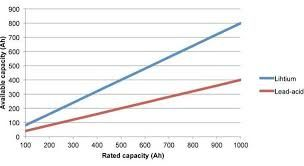•# WHAT IS BATTERY RATING AND HOW TO READ TECHNICAL INFORMATION

\$0.00 \$0.00

A battery's C (capacity) rating is the maximum safe continuous discharge rate of a battery.
A batteries maximum acceptable discharge rate is calculated by multiplying its current rating by it's C rating.
A C rating of 1 means the battery can be discharged at the same rate as its capacity.
So a 1 Amp (1000mah) battery with a Crating of 1 can be continuously discharged at 1 Amp.
A more typical example: 11.1 volt 2000mAh (2 amp) battery rated at 10C = 2 Amps x 10C = 20 amps continuous discharge.
You use the C rating to determine if a given battery has sufficient current available to drive your motor(s) at maximum output.
Exceeding the C rating for a significant period will result in damage to the battery and can cause it to burn or even explode.
Batteries also often indicate a second (safe) short term maximum C rating (commonly 50% over the continuous C rating).
A typical LiPo battery rated at 3S1P 10,000mAh 20C can be broken down as follows:
3S1P means 3 cells in series none in parallel, since individual cells are 3.7 volts, the battery is 11 volts.
10,000mAh means since the cells are only in series each 3.7 volt cell has 10,000mAh or 10 Amp Hours of capacity.
the 20C rating means the Battery can be discharged at a rate 20 times its 10 Amp Hour capacity up to 200 Amps continuou